Cuisenaire rods are physical mathematical learning supports in which each colour rod represents a different number.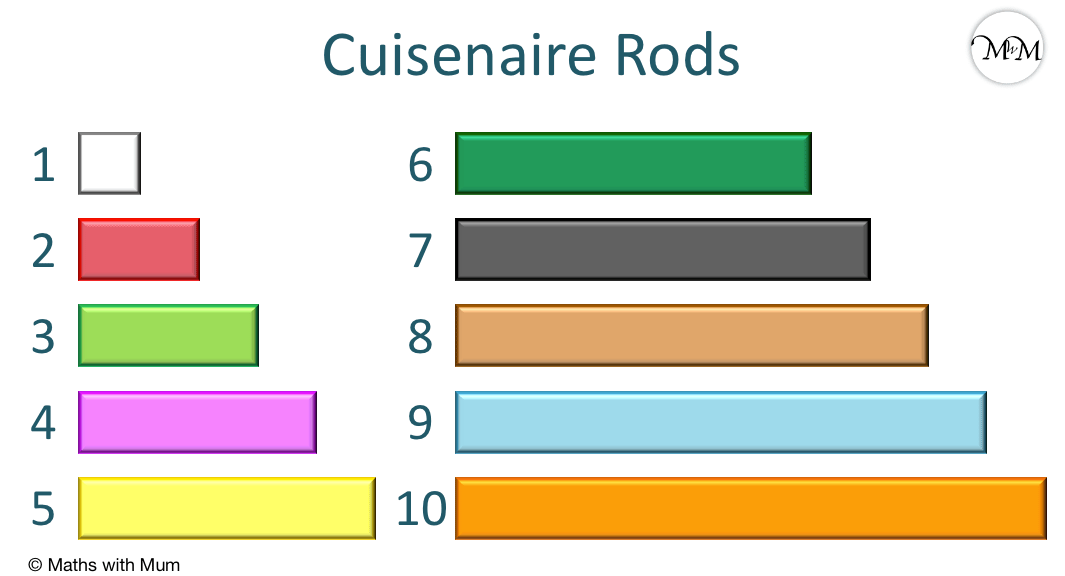• The lengths of each colour Cuisenaire rod is shown in the chart above. The white rod is 1 cm long.
• Each colour rod is worth a different value.
• Cuisenaire rods are useful for students to build their number sense as the rods allow for easy comparison between each length.
• The rods can also be used to build understanding of the concepts of addition, subtraction, multiplication, division, fractions, decimals and ratio.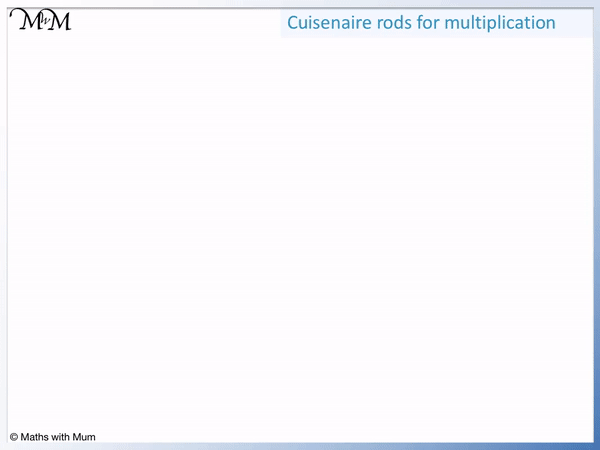• Cuisenaire rods can be used to teach multiplication as a repeated addition process.
• We can line up Cuisenaire rods to show that many smaller quantities are equivalent to one larger quantity.
• For example we can see that five lots of the 2 rods are equivalent in length to one 10 rod.
• This can help to reinforce the conceptual understanding of 2 × 5 = 10 and other multiplication facts.Supporting Lessons# Cuisenaire Rods

## What are Cuisenaire Rods?

Cuisenaire rods are coloured plastic bars of different lengths, which are used to represent different number sizes. These rods are used to demonstrate the comparative sizes of numbers and can be useful in teaching basic addition, subtraction, multiplication and division. It is also possible to use Cuisenaire rods to demonstrate equivalent fractions, decimal numbers and ratio.

Here is a colour chart showing the different Cuisenaire rod values.Cuisenaire rods were invented by Belgian schoolteacher Emile-Georges Cuisenaire in the 1950s.

They are often used in primary schools for teaching number facts and therefore are commonly used with children between the ages of 4-7. However, they are often used with children older than this who struggle with mathematics as they can support their understanding of number size. Cuisenaire rods can also be used to teach older children between 11-16 concepts such as ratios and fractions.

## How to Introduce Cuisenaire Rods

Cuisenaire rods can be introduced by asking for the rods to be placed in order of size and then asking if any smaller rods can be combined to make the same length as the larger rods. Then ask if further combinations of rods that are of equal lengths can be found. Can we make any number using only white or red rods? These activities will help build the idea of equivalences and familiarise children with the rod values.

Showing basic equivalences can be helpful such as this example of 2 + 3 = 5. Can your child find further combinations of rods that are equal in size?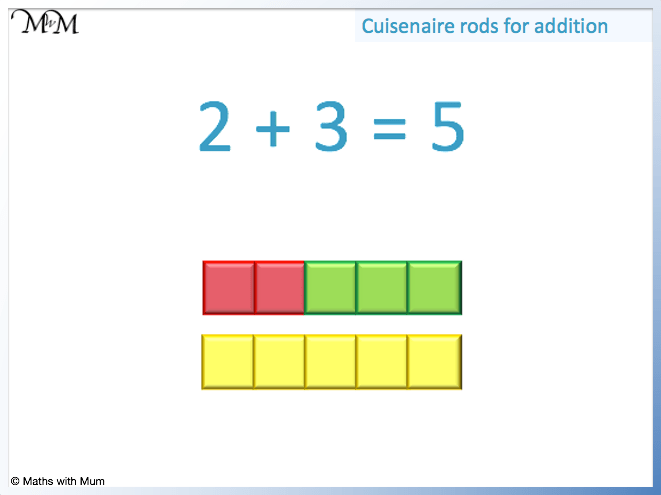Further introductory Cuisenaire rod ideas are:

• Can you make a combination of rods the same length as the blue rod using exactly 3 rods?
• Can you make a rod the same length as the orange rod using only red rods?
• How about using only green rods?
• What about using only red and green rods?
• What is larger, a black plus a white or a brown plus a purple?

Games that ask children to create equivalent lengths and to play with the size of different rods are useful for introducing Cuisenaire rods.

## Why Use Cuisenaire Rods

Cuisenaire rods are used because they are a simple and hands-on way to compare number sizes. They help to engage children in mathematics as they are brightly coloured and easy to stack together. Cuisenaire rods also have a number of purposes across many different branches of mathematics which makes them a versatile tool.

Here are some of the advantages and disadvantages of teaching with Cuisenaire rods.

They provide tactile learning for students with maths difficulties You cannot count up in ones on each block
Helps to build comparative number sense The rods can be limited to teaching smaller numbers
Different coloured rods for each size Lengths are not written on the rods
Can be used in a variety of games/settings Cannot represent all fractions easily
Simple to use Potentially limited in challenge
Allows for trial and error without fear of making mistakes Limited feedback on learning
Time to explore numbers without rushing
Can build stronger understanding of numbers using visual learning

## What are the Values of the Cuisenaire Rods?

Cuisenaire rods always have the same fixed values:

Cuisenaire Rod Colour Colour Code Value Length
White w 1 1 cm
Red r 2 2 cm
Lime Green g 3 3 cm
Purple p 4 4 cm
Yellow y 5 5 cm
Dark Green d 6 6 cm
Black b 7 7 cm
Tan (Brown) t 8 8 cm
Blue B 9 9 cm
Orange o 10 10 cm## How to Use Cuisenaire Rods to Teach Addition

To teach addition using Cuisenaire rods, combine two smaller rods end to end and place them alongside a larger rod that is the same length as the two smaller rods combined. For example 2 white rods are the same length as 1 red rod. We can say that 1 + 1 = 2 because they are the same length. This can then be extended to larger numbers by combining two or more larger rods.

For example, the red plus green rods are the same length as the yellow rod.

Since the red = 2, the green = 3 and the yellow = 5, we can see that 2 + 3 = 5.Here is a larger example of 4 + 6 = 10 using the purple, dark green and orange rods.Cuisenaire rods are great for teaching the number bonds to 10. These are the pairs of numbers that add to make 10.

Simply ask your child to find and list as many different combinations of rods that add to make the same length as the orange 10 rod.

## How to Use Cuisenaire Rods to Teach Subtraction

To teach subtraction using Cuisenaire rods, find a combination of two smaller rods that are the same length as one larger rod. Place the two smaller rods alongside the larger rod to show that they are the same size. Then remove the rod that you are subtracting and the remaining rod is the answer. Cuisenaire rods help to reinforce that the subtraction gives us the difference between two numbers.

For example, here is 7 – 4 = 3.

The black rod is worth 7, the purple is worth 4 and the lime green is worth 3.To show the subtraction of 4, simply remove the purple 4 rod. The remaining green rod is the answer. This shows us that 3 is the difference between 7 and 4.

Here is another example of subtraction with Cuisenaire rods. We have 9 – 2 = 7.The blue is worth 9, the red is worth 2 and the black is worth 7.

Removing the red 2 rod leaves us with the red 7 rod. 7 is the difference between 9 and 2.

## How to Use Cuisenaire Rods to Teach Multiplication

Cuisenaire rods can be used to teach multiplication by combining rods of the same size end-to-end to make a larger value. The number of rods combined together tell us how many lots of the base number we have. For example, 2 × 5 = 10 can be shown by lining up 5 lots of the red size 2 rods alongside an orange size 10 rod.We can see that 5 lots of 2 is equal to 10.

Here is an introductory example of 1 × 4 = 4.

Each white Cuisenaire rod is worth 1 and the purple Cuisenaire rod is worth 4. We can see that 4 lots of 1 is the same as 4.Cuisenaire rods can be used to build a conceptual understanding of multiplication as repeated addition.

## How to Use Cuisenaire Rods to Teach Division

Cuisenaire rods can be used to teach division by first combining rods of the same size end-to-end to form a rod of a larger size. The smaller rods can then be counted to see how many go into the larger rod. The number of smaller rods is the answer to the division. For example, a blue size 9 rod can be made from 3 lime green size 3 rods. This demonstrates the division of 9 ÷ 3 = 3.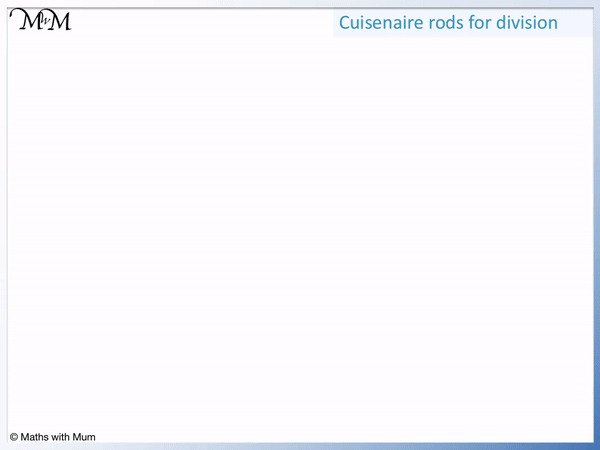There are 3 lots of the smaller lime green rods and so, the answer to the division is 3.

Here is another example of division using Cuisenaire rods. We have 6 ÷ 1 = 6.

The dark green rod is worth 6. The white rod is worth 1.

There are 6 white rods and so, 6 ÷ 1 = 6.## How to Use Cuisenaire Rods to Teach Fractions

To teach fractions using Cuisenaire rods, a smaller rod can be used to represent the numerator and the larger rod can be used to represent the denominator. For example a size 2 red rod and a size 6 dark green rod represent the fraction 2/6. We can see that the red rod is one third of the size of the dark green rod and so, 2/6 is the same as 1/3.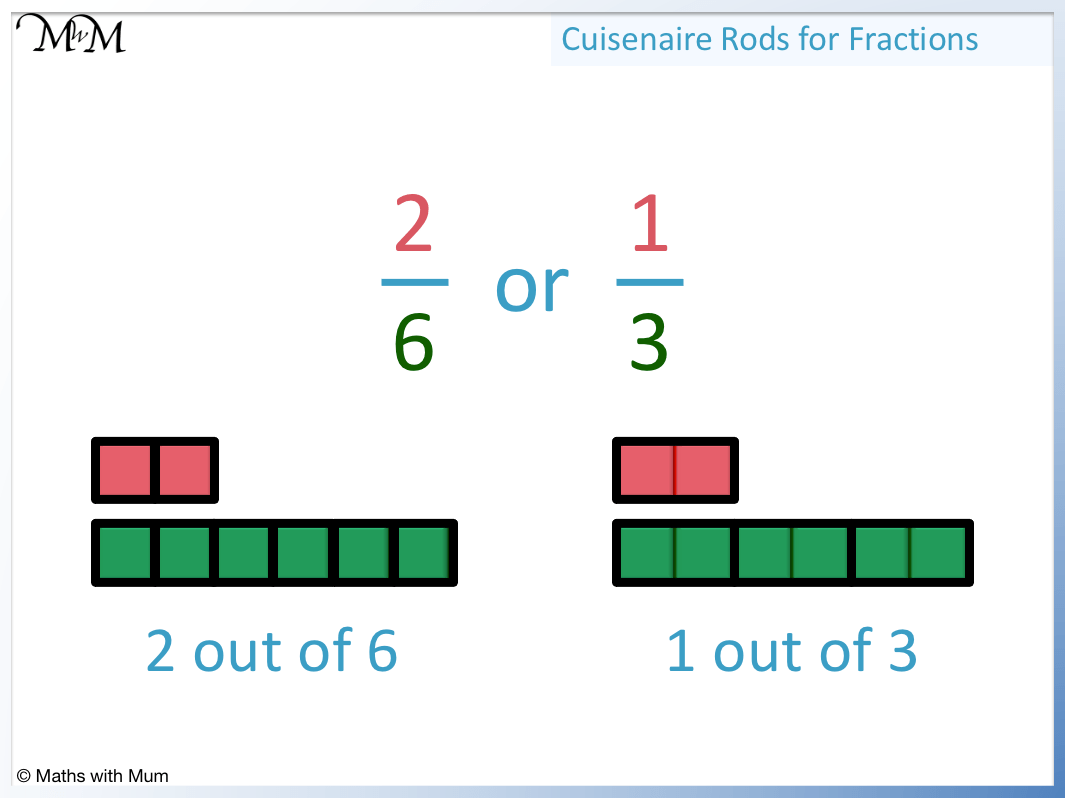The number of times the smaller rod fits into the larger rod tells us the simplified fraction.

Cuisenaire rods are useful to build basic fraction understanding, such as comparing a size 5 yellow with a size 10 orange rod can easily show that 5 is half of ten.

## How to Use Cuisenaire Rods to Teach Decimals

Cuisenaire rods can be useful tools for explaining the comparative sizes of decimals. For example, labelling the size 10 orange rod as worth 1, then the size 1 white rod will be one tenth of this, which is 0.1. Then the red size 2 rod is worth 0.2 and so on. Decimals can be taught by combining Cuisenaire rods to make further decimal numbers.

Here the orange rod is worth one whole. This means that every white size 1 rod is worth 0.1.

We can combine 3 white rods to make 0.3.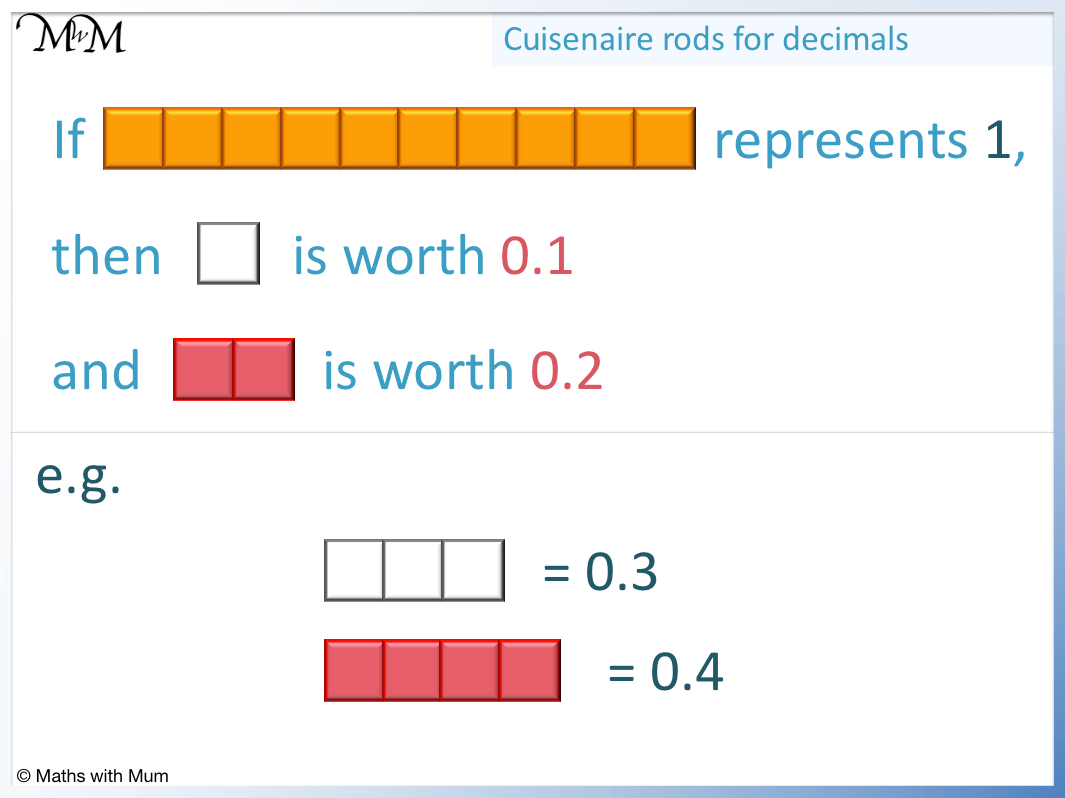Alternatively, two red size 2 rods would be worth 0.4 because this is the same length as 4 of the 0.1 rods.

Cuisenaire rods are very useful when teaching the comparative sizes of decimals. In the diagram above we can easily see that 0.4 is just under half of the size of 1 whole.

We can also represent decimals in other ways if necessary. For example, this time the yellow size 5 rod is worth one whole.

This means that each size 1 white rod is one fifth, or 0.2.

Every time we add a white rod, we count up in 0.2s.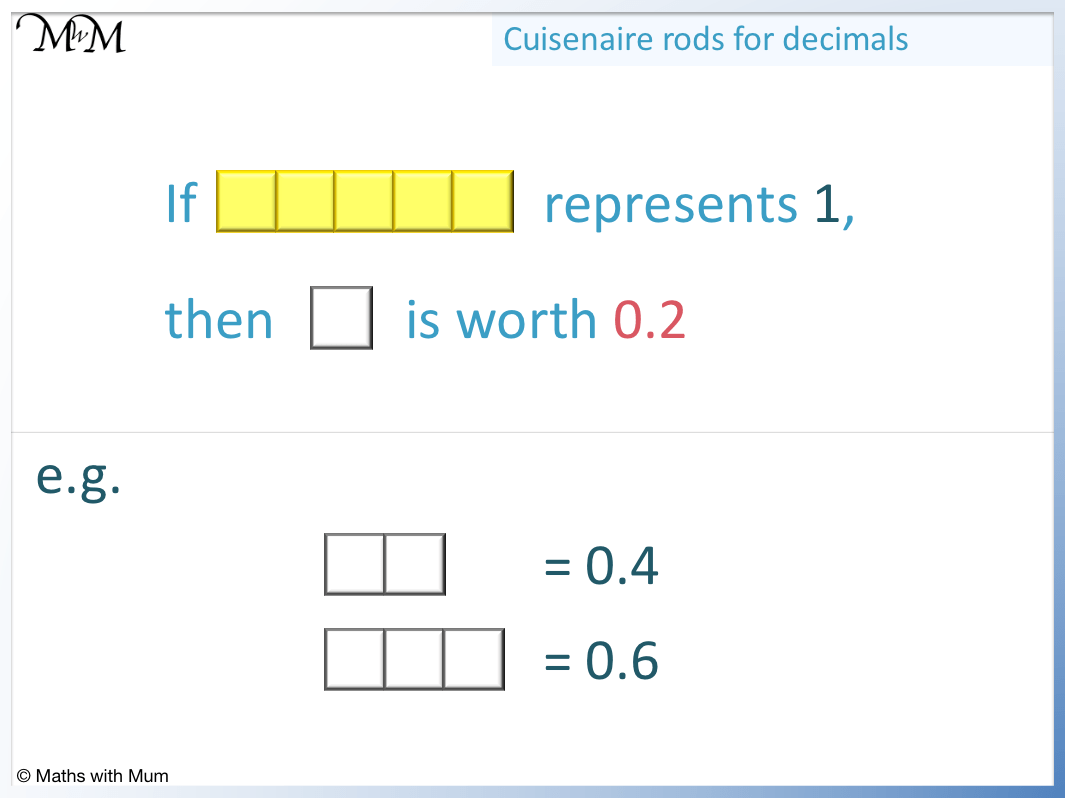## How to Use Cuisenaire Rods to Teach Ratio

Due to the ease in which Cuisenaire rod sizes can be compared, they are a very useful tool for teaching ratios. By allocating a value to the smallest white rod, larger values can be demonstrated. For example, if the white size 1 rod is worth 5, then the red size 2 rod is worth 10.

The red rod is always double the value of the white rod, no matter what the white rod is worth.Here is another example of teaching ratio using Cuisenaire rods. Here the lime green size 3 rod is worth 30.

Because 3 white rods make 1 lime green rod, the white rod must be worth 10.We can then see that the yellow rod must be worth 50 because we have 5 lots of the white rods, which are worth 10 each.

Here we have a purple rod worth 80 and we want to know the value of the red rod. We first see that 4 white rods go into the purple rod, so each white rod must be worth 20.

The red rod is always double the white rod and so, it is worth 40.## Cuisenaire Rod Activities

Here are a list of short Cuisenaire rod activities:

• Put the Cuisenaire rods in order of size from smallest to largest to make a ‘staircase’.
• Count how many white rods are needed to make the other size rods.
• Find which colour rods can be make using only the red rods.
• Find as many combinations of two smaller rods which are the same length as one larger rod.
• Find as many combinations of three smaller rods which are the same length as one larger rod.
• Find as many different pairs of rods that have a difference in size of 1.
• Find a pair of rods that have a difference of exactly 6.
• Use the rods to find and list all different combinations of rods that add to make 10.
• Cuisenaire rod snap – take it in turns to draw a different rod from your pile. If the two rods add to make 10, the person who says snap first wins the rods.Now try our lesson on Place Value with Base Ten Blocks where we learn how to use base ten blocks.